IF YOU CAN SEE THIS go to /sfa/Nuprl/Shared/Xindentation_hack_doc.html

### Functions

"Function" and "Type" are the fundamental a priori concepts of type theory. Nuprl's computational type theory is a constructive semantical theory about notations adequate for an intuitionistic account of functions, and the Nuprl logic emphasizes the computational aspect of notation.

Notations in Nuprl are "grounded" computationally. A class of computationally significant expressions is presupposed by the semantics of denotation (see Computation). It should be emphasized that the relation of a computional expression to the result of executing it is not the same as that expression standing for an abstract value, even though we sometimes call executing an expression "evaluation".
One defines a method for notating a class of values, i.e. a "type" (see Types), by specifying when two notations stand for the same value of that type. These notations are taken from the presupposed computational language, and it is required that whenever two expressions are "computationally equivalent" then if either stands for a value in some type, both stand for the same value.

For example,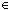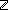, therefore 0 = if 1=2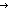3 ; 0 fisince executing "if 1=23 ; 0 fi" results in the expression "0".
Indeed, radically, "0 = if 1=2X ; 0 fi" is true for any closed expression X, such as the non-integer "<0,0>" or even the non-evaluable expression "(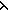x.x(x))(x.x(x))". The fact that these are provable in Nuprl is an aspect of taking the computational basis for notation seriously.

As in normal practice, the most common way of expressing functions is by means of expressions containing variables. By substituting constants for those variables, the resulting expression stands for the value of the function on the values those constants stand for.
For example, "x+1" stands (in the variable x) for the successor function on.
A basic constraint on the computational behavior of such an attempt to express a function is that if one replaces an argument expression by an "equal" one, then the resulting full expressions are also "equal". This "equality" of notations is dependent on type, on both the input and output types in the case of functions.
Consider the expression "if x=01 ; x fi". Although it expresses (in x) a function fromto, it does not express a function from (mod 2) tobecause when applied to "0" it evaluates to "1", but when applied to "2", which stands for the same value of (mod 2) as "0", it evaluates to "2", which is not equal to "1" in, of course. Another, trivial, example of such an expression is simply "x" which obviously expresses the identity function, in x, over any type, but does not express a function from (mod 2) tosince 0 = 2mod 2 but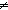2.

The "Abstraction" Operator:x.b(x)

It is notationally quite handy to have a form for expressing functions with closed expressions, i.e. without free variables, and a notation for applying functions to arguments in which the notation for the function can be separated from the argument. We can then build a type of such functional expressions for any given input and output types.

Our two-place notation for function application is "f(t)" where "f" indicates the place for the function expression, and "t" indicates the place for the argument expression. We mediate the two methods for expressing functions (viz. expressions with free variables, versus closed function expressions applied via "<fun>(<arg>)") with the so-called "abstraction" operator "x.b(x)".
For example, the doubling function on integers expressed as "x+x" using free variables as arguments, may be expressed in closed form as "x.x+x".
Naturally, the expression "(x.x+x)(2)" evaluates according to the rewrite sequence:

(x.x+x)(2) *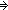2+2 *4

Generally, "(x.b(x))(t)" is evaluated by evaluating "b(t)".

Function Types are collections of these closed forms expressing functions.